Power Functions Click here to open an associated Mathcad worksheet: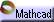Graphic Representation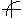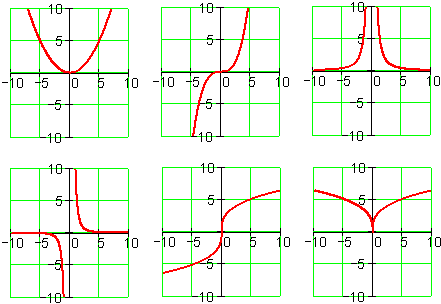Power functions take many forms.

Graphs of  y = a x b  are simple forms of polynomials when the power is a positive integer. They are simple forms of rational functions when the power is a negative integer.

Fractional powers provide further forms.

Points where power functions exhibit specific behaviors are described using terminology such as:

• Singularity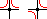• Inflection Point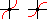• Cusp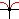The above graphs show just a few of the members of the family of power functions. To see how these and other power graphs can be created by tuning the family's parameters, see the associated worksheet.